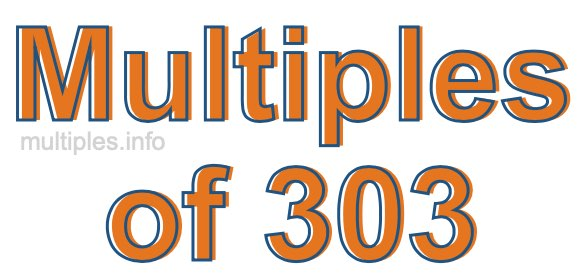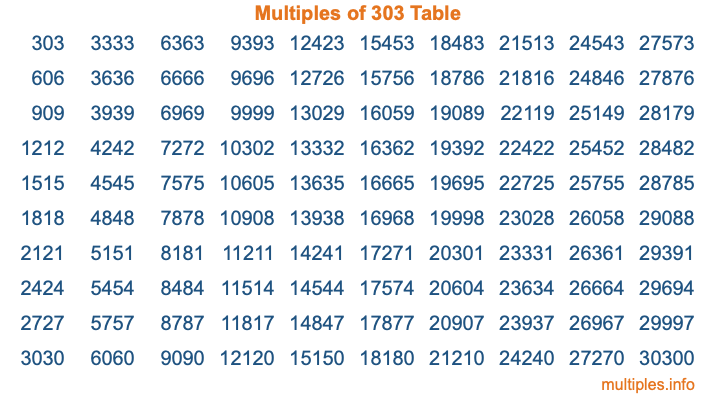Multiples of 303Welcome to the Multiples of 303 page. Here we will first teach you everything you will ever need to know about the multiples of 303, and then give you a study guide summary of everything we taught you to make sure you remember it all. Use this page to look up facts and learn information about the multiples of 303. This page will make you a multiples of three hundred three expert!

Definition of Multiples of 303
Multiples of 303 are all the numbers that when divided by 303 equal an integer. Each of the multiples of 303 are called a multiple. A multiple of 303 is created by multiplying 303 by an integer.

Therefore, to create a list of multiples of 303, you start with 1 multiplied by 303, then 2 multiplied by 303, then 3 multiplied by 303, and so on for as long as you want. Thus, the list of the first five multiples of 303 is 303, 606, 909, 1212, and 1515. To see a larger list of multiples of 303, see the printable image of Multiples of 303 further down on this page. We also have a category where you can choose any nth multiple of 303.

Multiples of 303 Checker
The Multiples of 303 Checker below checks to see if any number of your choice is a multiple of 303. In other words, it checks to see if there is any number (integer) that when multiplied by 303 will equal your number. To do that, we divide your number by 303. If the the quotient is an integer, then your number is a multiple of 303.

Is  a multiple of 303?

Least Common Multiple of 303 and ...
A Least Common Multiple (LCM) is the lowest multiple that two or more numbers have in common. This is also called the smallest common multiple or lowest common multiple and is useful to know when you are adding our subtracting fractions. Enter one or more numbers below (303 is already entered) to find the LCM.

Check out our LCM Calculator if you need more details about the Least Common Multiple or if you need the LCM for different numbers for adding and subtraction fractions.

nth Multiple of 303
As we stated above, 303 is the first multiple of 303, 606 is the second multiple of 303, 909 is the third multiple of 303, and so on. Enter a number below to find the nth multiple of 303.

th multiple of 303

Multiples of 303 vs Factors of 303
303 is a multiple of 303 and a factor of 303, but that is where the similarities end. All postive multiples of 303 are 303 or greater than 303. All positive factors of 303 are 303 or less than 303.

Below is the beginning list of multiples of 303 and the factors of 303 so you can compare:

Multiples of 303: 303, 606, 909, 1212, 1515, etc.

Factors of 303: 1, 3, 101, 303

As you can see, the multiples of 303 are all the numbers that you can divide by 303 to get a whole number. The factors of 303, on the other hand, are all the whole numbers that you can multiply by another whole number to get 303.

It's also interesting to note that if a number (x) is a factor of 303, then 303 will also be a multiple of that number (x).

Multiples of 303 vs Divisors of 303
The divisors of 303 are all the integers that 303 can be divided by evenly. Below is a list of the divisors of 303.

Divisors of 303: 1, 3, 101, 303

The interesting thing to note here is that if you take any multiple of 303 and divide it by a divisor of 303, you will see that the quotient is an integer.

Multiples of 303 Table
Below is an image of the first 100 multiples of 303 in a table. The table is in chronological order, column by column. The first column has the first ten multiples of 303, the second column has the next ten multiples of 303, and so on.The Multiples of 303 Table is also referred to as the 303 Times Table or Times Table of 303. You are welcome to print out our table for your studies.

Negative Multiples of 303
Although not often discussed or needed in math, it is worth mentioning that you can make a list of negative multiples of 303 by multiplying 303 by -1, then by -2, then by -3, and so on, to get the following list of negative multiples of 303:

-303, -606, -909, -1212, -1515, etc.

Multiples of 303 Summary
Below is a summary of important Multiples of 303 facts that we have discussed on this page. To retain the knowledge on this page, we recommend that you read through the summary and explain to yourself or a study partner why they hold true.

There are an infinite number of multiples of 303.

A multiple of 303 divided by 303 will equal a whole number.

303 divided by a factor of 303 equals a divisor of 303.

The nth multiple of 303 is n times 303.

The largest factor of 303 is equal to the first positive multiple of 303.

303 is a multiple of every factor of 303.

303 is a multiple of 303.

A multiple of 303 divided by a divisor of 303 equals an integer.

303 divided by a divisor of 303 equals a factor of 303.

Any integer times 303 will equal a multiple of 303.

Multiples of a Number
Here you can get the multiples of another number, all with the same attention to detail as we did for multiples of 303 on this page.

Multiples of
Multiples of 304
Did you find our page about multiples of three hundred three educational? Do you want more knowledge? Check out the multiples of the next number on our list!